Dynamics

Dynamics is all about the forces acting on objects and their resultant movements.

Newton's laws of motion

Sir Isaac Newton came up with several physical laws that relate forces and motion.

Newton's first law states that an object will remain in equilibrium as long as the resultant of the forces acting on it is zero.

That does not mean that the object is stationary, it means that it stays at a constant velocity, which can be zero.

Newton's second law states that the resultant force on an object is equal to its rate of change of momentum, which for objects with constant mass is simplified down to

$$\sum \mathbf{F} = m \mathbf{a}$$

An object's momentum is defined as the product of its mass and velocity $$\mathbf{p} = m\mathbf{v}$$ And the resultant force is equal to the first time-derivative of momentum according to N2L \begin{align} \sum \mathbf{F} &= \frac{d\mathbf{p}}{dt} \\ &= \frac{d \left( m \mathbf{v} \right)}{dt} \end{align} But if an object's mass stays constant over time, which is basically true in most real-life cases \begin{align} \sum \mathbf{F} &= m\frac{d\mathbf{v}}{dt} \\ &= m\mathbf{a} \end{align}

Where $m$ is the object's mass in kilograms, and $\mathbf{a}$ is its acceleration in $\textrm{ms}^{-2}$. Applying force on an object causes it to accelerate.

Bear in mind that the resultant force and the acceleration are always in the same direction.

Newton's third law states that for every action there is always opposed an equal reaction.

That means that pushing an object with a force causes an equal and opposite force from the object to your hand. It is the reaction that you feel on your hand when you push something.

Example

Q) A man drags a 20kg box attached to a rope across a rough surface at an angle of 14° to the horizontal. If the surface has a coefficient of friction of 0.5, what is the minimum force he must exert to move the box? The rope can be modelled as a light inextensible string.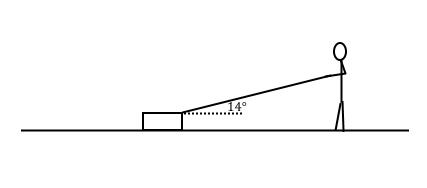A) The weight of the box is $20g = 196\textrm{N}$. Since the rope is an inextensible string, we can say that the tension in the rope $T$ is equal to the force the man must exert. We're also told that $\mu = 0.5$.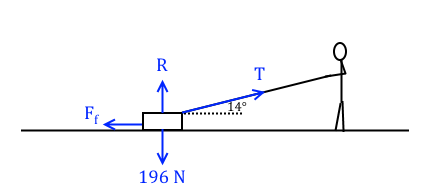Taking horizontal equilibrium

$$F_f - T\cos(14^{\circ}) = 0$$

$$\therefore F_f = T\cos(14^{\circ})$$

Taking vertical equilibrium

$$T \sin(14^{\circ}) + R - 196 = 0$$

But we have $F_f = 0.5R$ and $F_f = T\cos(14^{\circ})$, so sub $R = 2T\cos(14^{\circ})$ into the equation above

$$T \sin(14^{\circ}) + 2T\cos(14^{\circ}) - 196 = 0$$

$$T(\sin(14^{\circ})+2\cos(14^{\circ})) - 196 =0$$

$$\therefore T = \frac{196}{\sin(14^{\circ})+2\cos(14^{\circ})} = 89.8 \textrm{N} \left(\textrm{3.s.f.}\right)$$

So to overcome friction on the box he must exert a force of at least 89.8N (3.s.f.) on the rope.

Example

Q) A 5kg particle is released from rest and slides down a slope inclined at 45° to the horizontal. It travels 4m before hitting the bottom of the slope. If the coefficient of friction of the slope is $\frac{1}{4}$, what is the velocity of the particle the instant before it hits the bottom of the slope?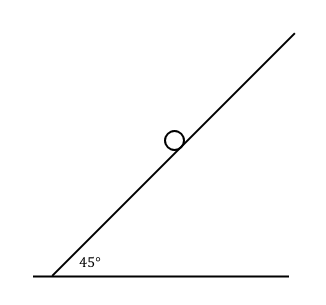A) The weight of the particle is $5g = 49\textrm{N}$, and the coefficient of friction for the slope is $\frac{1}{4}$. We know that the particle is moving down the plane and travels 4m before it hits the bottom of the slope.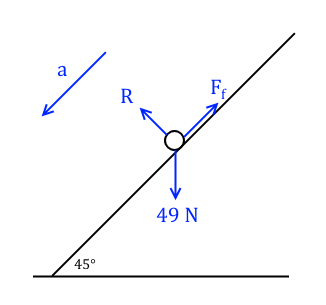Firstly take equilibrium perpendicular to the slope to find the reaction force $R$, since the particle isn't moving in that direction

$$R - 49\cos(45^{\circ}) = 0$$

$$\therefore R = 49\cos(45^{\circ}) = 34.6\textrm{N} \left(\textrm{3.s.f.}\right)$$

We were given that $\mu = \frac{1}{4}$ so $F_f = \frac{1}{4}\times 49\cos(45^{\circ}) = 8.66\textrm{N}$ (3.s.f.). Then resolve forces parallel to the slope, using N2L $\left(\sum F=ma\right)$.

$$49 \cos(45^{\circ}) - F_f = 5a$$

$$49 \cos(45^{\circ}) - 8.66 = 5a$$

$$\therefore a = \frac{49 \cos(45^{\circ}) - 8.66}{5} = 5.20 \textrm{ms}^{-2} \left(\textrm{3.s.f.}\right)$$

The particle was released from rest so $u = 0$, and $s = 4\textrm{m}$. We want to find $v$ so we will use $v^{2}=u^{2}+2as$.

$$v^{2}=u^{2}+2as$$

$$v^{2}=0 + \left(2\times 5.2 \times 4\right)$$

$$\therefore v = \sqrt{\left(2\times 5.2 \times 4\right)} = 6.45\textrm{ms}^{-1} \left(\textrm{3.s.f.}\right)$$

Connected particles

Sometimes we have particles connected at either end of a light inextensible string. Light inextensible strings are modelled as having no significant mass and being inelastic. That means that tension throughout the string is always the same, and any acceleration for both particles is always the same too.

Tow bars and horizontal strings

In any situation where one object pulls another object, we can model them as two particles connected by a light inextensible string.

Example

Q) A truck of mass 2,000kg is towing a car of mass 800kg. The truck's engine exerts a constant force of 5.2kN to move both itself and the car forward. The sum of the frictional forces acting on the truck is 2.3kN and the sum of the frictional forces acting on the car is 400N. If the truck has a forward velocity of $23\textrm{ms}^{-1}$, find its velocity after five seconds. The towbar may be modelled as a light inextensible string.A) The towbar is modelled as a light inextensible string, so the tension in the string and the acceleration in the whole system remain constant.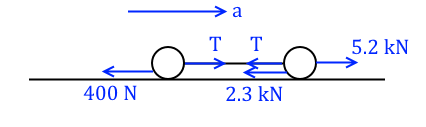Resolving forces acting on the car and using N2L

$$T - 400 = 800a$$

Resolving forces acting on the truck and using N2L

$$5200 - 2300 - T = 2000a$$

$$\Rightarrow 2900 - T = 2000a$$

We now have two simultaneous equations. Adding them together, eliminating $T$, we find

$$2900-400 = 2800a$$

$$\therefore a = \frac{2500}{2800} = 0.892 \textrm{ms}^{-2} \left(\textrm{3.s.f.}\right)$$

The initial forward velocity of the truck is $23\textrm{ms}^{-1}$, $a = 0.892 \textrm{ms}^{-2}$, and $t=5\textrm{s}$. Therefore we can use $v=u+at$.

\begin{align} v &= 23 + \left(0.892\times 5\right) \\ &= 27.5 \textrm{ms}^{-1} \left(\textrm{3.s.f.}\right) \end{align}

Pulleys

Sometimes we have particles connected at either end of a light inextensible string, but the string goes around a smooth pulley. A smooth pulley exerts no frictional forces on the string as either particle moves.

In an exam you might also be asked to calculate the resultant force on the pulley. This is simply the resultant of the tension forces in the string. I will show you how to calculate this in one of the exams below.

Example

Q) Two particles both attached to a light inextensible string hang vertically on either side of a pulley. Particle $A$ has mass 5kg and particle $B$ has mass 10kg. They are held in equilibrium, and then released so that particle $B$ begins to fall downwards. After one second particle $B$ hits the ground. How high off the ground was particle $B$ before it was released?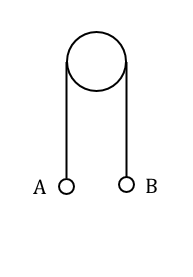A) The weight of particle $A$ is $5g = 49\textrm{N}$ and the weight of particle $A$ is $10g = 98\textrm{N}$. The tension is constant throughout the string, and the acceleration of both particles is also constant. We are told that particle $B$ moves downwards, so particle $A$ therefore moves upwards.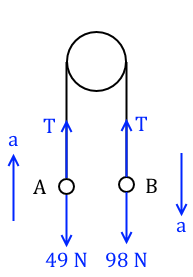Resolving forces acting on $A$ vertically and using N2L

$$T - 49 = 5a$$

Resolving forces acting on $B$ vertically and using N2L

$$98 - T = 10a$$

We now have two simultaneous equations. Adding them together, eliminating $T$, we find

$$49 = 15a$$

$$\therefore a = \frac{49}{15} = 3.26 \textrm{ms}^{-2} \left(\textrm{3.s.f.}\right)$$

The time taken for $B$ to hit the ground was one second, and the particles were released from rest so $u = 0$. So to find $s$ we'll use $s = ut+\frac{1}{2}at^{2}$.

$$s = 0 + \left(\frac{1}{2}\times 3.26 \times 1\right)$$

$$\therefore s = 1.63 \textrm{m} \left(\textrm{3.s.f.}\right)$$

Example

Q) (Hard) Two particles both attached to a light inextensible string are placed either side of a pulley at the top of a rough slope. Particle $A$ has mass 2kg and sits on the slope, and particle $B$ has mass $m$ and hangs vertically from the pulley, held 4m above the ground. The slope is 35° from the horizontal and has coefficient of friction 0.3. Given that when the particles are released from rest particle $B$ moves downwards and hits the ground after three seconds, find the value of $m$ and show that the resultant force on the pulley is 40N.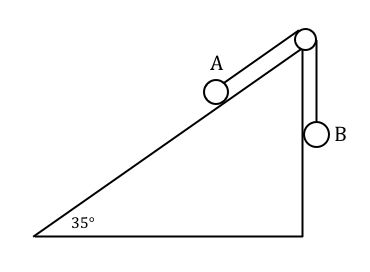A) Let's look at the motion of $B$ first to find the acceleration in the system. The particles are released from rest, so $u=0$, and we are given $s=4\textrm{m}$ and $t=3\textrm{s}$. So we'll use $s = ut+\frac{1}{2}at^{2}$, rearranging for $a$.

$$a = \frac{2s}{t^{2}} = \frac{8}{9} = 0.889 \textrm{ms}^{-2} \left(\textrm{3.s.f.}\right)$$

After the particles are released from rest, particle $B$ moves downwards, therefore particle $A$ moves upwards and the friction against $A$ acts down the slope.

The weight of particle $A$ is $2g = 19.6\textrm{N}$ and the weight of particle $B$ is $mg$. They are attached by a light inextensible string so the tension throughout the string is constant and so is the acceleration of both the particles.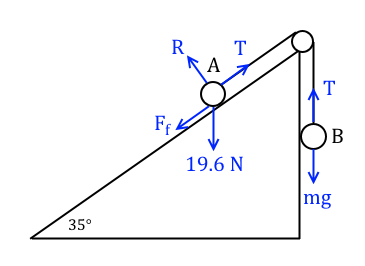Now to resolve forces. Resolving the forces acting on particle $B$ vertically and using N2L

$$mg - T = \frac{8}{9}m$$

Resolving forces acting on particle $A$ perpendicular to the slope and taking equilibrium

$$R - 2g\cos(35^{\circ}) = 0$$

$$\therefore R = 16.1 \textrm{N} \left(\textrm{3.s.f.}\right)$$

We were given $\mu = 0.3$ so $F_f = 0.3R = 4.82\textrm{N}$ (3.s.f.). Resolving forces acting on particle $A$ parallel to the slope and using N2L

$$T - F_f - 2g\sin(35^{\circ}) = 2a$$

$$T - 4.82 - 2g\sin(35^{\circ}) = \frac{16}{9}$$

We now have two simultaneous equations

$$mg - T = \frac{8}{9}m$$

$$T - 4.82 - 2g\sin(35^{\circ}) = \frac{16}{9}$$

Add them together, eliminating $T$, to get

$$mg - 4.82 - 2g\sin(35^{\circ}) = \frac{8}{9}m + \frac{16}{9}$$

Solve for $m$

$$m\left(g-\frac{8}{9}\right) = 4.82 + 2g\sin(35^{\circ}) + \frac{16}{9}$$

$$\therefore m = \frac{4.82 + 2g\sin(35^{\circ})+ \frac{16}{9}}{g-\frac{8}{9}} \approx 2 \textrm{kg}$$

Going back to the second of the simultaneous equations, we have

$$T = 4.82 + 2g\sin(35^{\circ}) + \frac{16}{9} = 22.7\textrm{N} \left(\textrm{3.s.f.}\right)$$

Then to find the resultant force on the pulley we find the resultant of the tension forces acting either side of the pulley.Therefore the resultant force on the pulley is equal to

$$T\cos\left(\frac{55}{2}\right) + T\cos\left(\frac{55}{2}\right) = 2\times 22.7\times\cos\left(\frac{55}{2}\right) = 40.3 \approx 40 \textrm{N}$$